### Home > CALC > Chapter 12 > Lesson 12.1.2 > Problem12-24

12-24.
1. Multiple Choice: The graph below shows the growth of a population P of sea lions introduced near a remote island. A reasonable differential equation to model this growth is: Homework Help ✎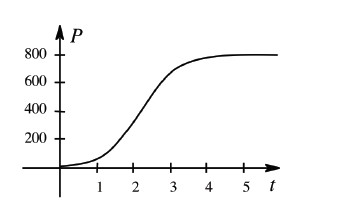1.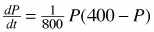2.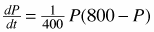3.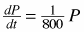4.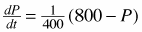5.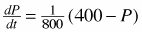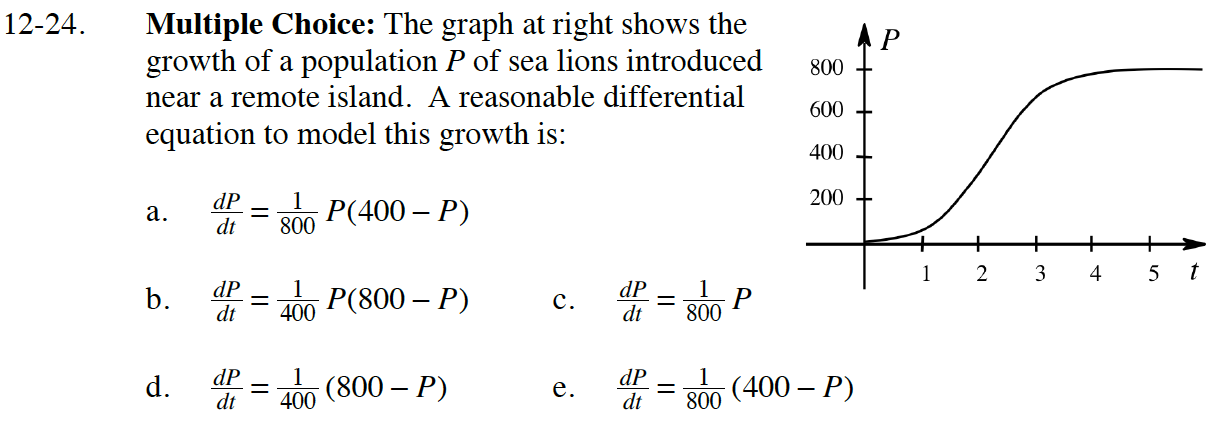By looking at the graph:

Which equation satisfies these conditions?